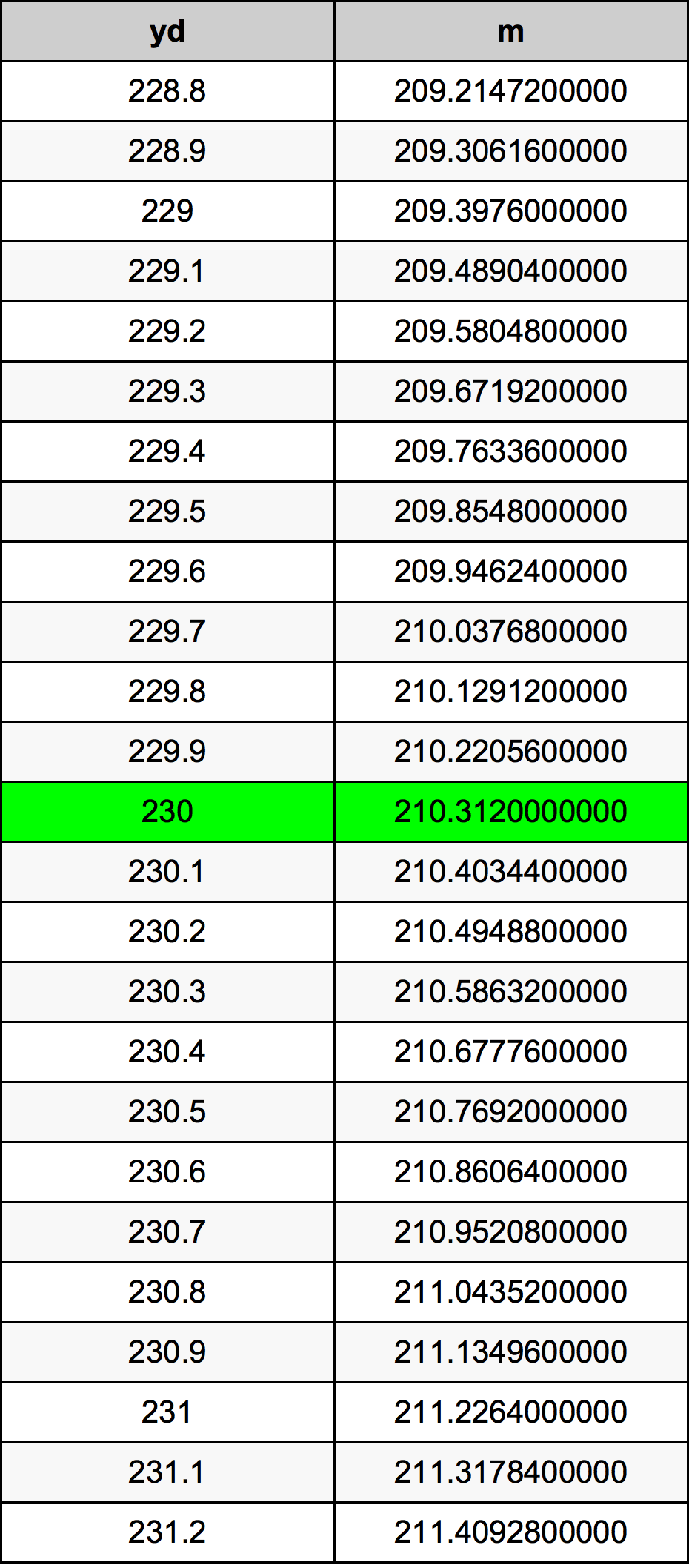Yards To Meters

# 230 yd to m230 Yards to Meters

yd
=
m

## How to convert 230 yards to meters?

 230 yd * 0.9144 m = 210.312 m 1 yd
A common question is How many yard in 230 meter? And the answer is 251.531058618 yd in 230 m. Likewise the question how many meter in 230 yard has the answer of 210.312 m in 230 yd.

## How much are 230 yards in meters?

230 yards equal 210.312 meters (230yd = 210.312m). Converting 230 yd to m is easy. Simply use our calculator above, or apply the formula to change the length 230 yd to m.

## Convert 230 yd to common lengths

UnitLengths
Nanometer2.10312e+11 nm
Micrometer210312000.0 µm
Millimeter210312.0 mm
Centimeter21031.2 cm
Inch8280.0 in
Foot690.0 ft
Yard230.0 yd
Meter210.312 m
Kilometer0.210312 km
Mile0.1306818182 mi
Nautical mile0.1135593952 nmi

## What is 230 yards in m?

To convert 230 yd to m multiply the length in yards by 0.9144. The 230 yd in m formula is [m] = 230 * 0.9144. Thus, for 230 yards in meter we get 210.312 m.

## 230 Yard Conversion Table## Alternative spelling

230 Yard to Meter, 230 Yard in Meter, 230 yd to Meters, 230 yd in Meters, 230 yd to m, 230 yd in m, 230 Yard to m, 230 Yard in m, 230 Yards to m, 230 Yards in m, 230 yd to Meter, 230 yd in Meter, 230 Yards to Meter, 230 Yards in Meter